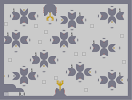### Get the nectarHover over the thumbnail for a full-size version.

Author NOBLE_TEMPLAR action author:noble_templar playable unrated 2007-03-18 2007-03-18 5 more votes required for a rating. \$Get the nectar#NOBLE_TEMPLAR#none#00000000<;000000000011000000000:=000000000011000<;000:<;5000000430:1000:=000;=:4000000:=001002<;=000;<000000:<;=0:003=:<000=:000000;=:<00000;<0000000000000;<000000=:000000000<;00=:00000000000000000:=00000000000000000000:<;50000000;1=000000000;=:400000001100000000000;<00000000:1<00000<;000=:0000000700000000:=00000000000:10004300:<;50000000000;1000:=00;=:400000000000600:<;=00;<000000<;0000000;=:<00=:000000:=00000000;<0000000000:<;=0000000=:0000000000;=:<00000000000000000000;<00000000000000000000052000000000000000<;000000000000000000000:=0000000000000<;00000:<;=00000<;00000:=00000;=:<00000:=0000:<;500000;<00000:<;=000;=:4000005200000;=:<0000;<00000000000000;<00000=:000000000000005200000000000000000000000000|5^192,420!5^216,324!5^432,204!5^564,252!5^480,84!5^432,396!5^48,204!5^720,444!5^84,60!5^720,60!12^324,240!12^384,204!12^444,240!12^492,120!12^432,84!12^432,156!12^612,96!12^672,60!12^732,96!12^624,252!12^564,288!12^624,324!12^672,444!12^612,480!12^672,516!12^480,396!12^480,468!12^420,432!12^324,360!12^264,324!12^204,360!12^144,420!12^204,456!12^144,492!12^156,240!12^96,204!12^36,240!12^84,96!12^204,96!12^144,132!9^84,456,0,0,4,23,1,0,0!9^540,432,0,0,4,23,1,0,0!9^732,480,0,0,4,23,1,0,0!9^264,396,0,0,4,23,1,0,0!9^96,276,0,0,4,23,1,0,0!9^144,60,0,0,4,23,1,0,0!9^384,276,0,0,4,23,1,0,0!9^372,120,0,0,4,23,1,0,0!9^672,132,0,0,4,23,1,0,0!9^684,288,0,0,4,23,1,0,0!11^36,564,300,72!2^264,300,0,1!2^348,360,-1,0!2^180,360,1,0!2^228,456,-1,0!2^144,396,0,1!2^144,516,0,-1!2^672,540,0,-1!2^228,96,-1,0!2^60,96,1,0!2^144,156,0,-1!2^96,180,0,1!2^180,240,-1,0!2^300,144,0,-1!12^336,72!12^336,60!12^336,48!12^264,72!12^264,60!12^264,48!12^264,48!0^300,84!0^300,84!0^300,96!0^300,90!0^300,84!0^300,78!0^306,102!0^312,108!0^318,114!0^318,120!0^312,120!0^312,102!0^318,108!0^294,102!0^288,102!0^288,108!0^282,108!0^282,114!0^282,120!0^288,120!2^336,120,1,0!2^672,168,0,-1!2^720,288,-1,0!2^768,480,-1,0!2^588,480,1,0!2^576,432,-1,0!2^624,348,0,-1!2^540,288,1,0!2^624,228,0,1!2^384,312,0,-1!2^300,240,1,0!2^384,180,0,1!2^468,240,-1,0!2^432,180,0,-1!2^516,120,-1,0!2^432,60,0,1!2^96,312,0,-1!2^48,456,1,0!2^480,492,0,-1!2^672,420,0,1!2^396,432,1,0!2^480,372,0,1!2^264,432,0,-1!11^36,516,132,576!9^360,540,0,0,6,23,1,-1,0!12^336,540!12^336,552!12^336,564!12^324,576!12^330,570!12^336,558!12^336,546!12^384,540!12^384,546!12^384,552!12^384,558!12^384,564!12^390,570!12^396,576!1^240,252!1^552,156!2^672,36,0,1!2^588,96,1,0!1^732,216!1^744,372!1^456,324!1^60,360!1^516,540!1^216,156!1^264,492!1^504,324!1^276,192!1^36,144!1^336,468!0^24,456!0^384,276!0^540,432!0^732,480!0^684,288!0^672,132!0^372,120!0^144,60!0^96,276!0^264,396!0^360,540!0^360,528!0^360,516!0^360,522!0^360,516!0^360,504!0^360,510!0^360,528!0^360,534!0^366,510!0^366,504!0^372,504!0^372,498!0^378,498!0^354,504!0^354,504!0^354,510!0^348,498!0^348,504!0^342,498!0^348,492!0^348,486!0^342,486!0^372,492!0^372,486!0^372,486!0^378,486!0^360,498!0^360,492!0^360,NaN!9^144,60,0,0,5,23,1,-1,0!9^144,60,0,0,4,23,1,0,0!9^144,60,0,0,5,23,1,-1,0!9^144,60,0,0,4,23,1,0,0!9^144,60,0,0,5,23,1,-1,0!9^144,60,0,0,4,23,1,0,0!0^144,60# This map is based on bees getting nectar (=6) dont go crying cos u cant beat it cos u can... hint get the switch with no gold first.... rawr